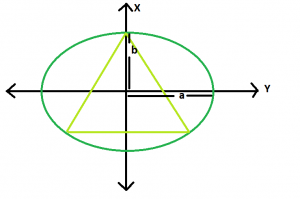# Largest triangle that can be inscribed in an ellipse

Given an ellipse, with major axis length 2a & 2b, the task is to find the area of the largest triangle that can be inscribed in it.

Examples:

```Input: a = 4, b = 2
Output: 10.3923

Input: a = 5, b = 3
Output: 10.8253
```

## Recommended: Please try your approach on {IDE} first, before moving on to the solution.Approach: So we know the ellipse is just the scaled shadow of a circle.Let’s find the scaling factor.

x^2/a^2 + y^2/b^2 = 1 is an ellipse. Rewrite this as:
(y*(a/b))^2+x^2 = a^2

This is just a vertically scaled down circle of radius a (think light falls from the top at an angle), and the vertical factor is a/b. The biggest triangle in the ellipse is then a scaled up version of the biggest triangle in the circle. Using a little geometry and taking symmetry into account, we can understand that the biggest such triangle is the equilateral one. It’s sides will be √3a and the area will be (3√3)a^2/4

Translating this to ellipse terms – we scale the horizontal dimension up by a factor a/b, and the area of the biggest triangle in the ellipse is,

A = (3√3)a^2/4b

Below is the implementation of above approach:

## C++

 `// C++ Program to find the biggest triangle ` `// which can be inscribed within the ellipse ` `#include ` `using` `namespace` `std; ` ` `  `// Function to find the area ` `// of the triangle ` `float` `trianglearea(``float` `a, ``float` `b) ` `{ ` ` `  `    ``// a and b cannot be negative ` `    ``if` `(a < 0 || b < 0) ` `        ``return` `-1; ` ` `  `    ``// area of the triangle ` `    ``float` `area = (3 * ``sqrt``(3) * ``pow``(a, 2)) / (4 * b); ` ` `  `    ``return` `area; ` `} ` ` `  `// Driver code ` `int` `main() ` `{ ` `    ``float` `a = 4, b = 2; ` `    ``cout << trianglearea(a, b) << endl; ` ` `  `    ``return` `0; ` `} `

## Java

 `//Java Program to find the biggest triangle ` `//which can be inscribed within the ellipse ` ` `  `public` `class` `GFG { ` ` `  `    ``//Function to find the area ` `    ``//of the triangle ` `    ``static` `float` `trianglearea(``float` `a, ``float` `b) ` `    ``{ ` ` `  `     ``// a and b cannot be negative ` `     ``if` `(a < ``0` `|| b < ``0``) ` `         ``return` `-``1``; ` ` `  `     ``// area of the triangle ` `     ``float` `area = (``float``)(``3` `* Math.sqrt(``3``) * Math.pow(a, ``2``)) / (``4` `* b); ` ` `  `     ``return` `area; ` `    ``} ` ` `  `    ``//Driver code ` `    ``public` `static` `void` `main(String[] args) { ` `     `  `        ``float` `a = ``4``, b = ``2``; ` `         ``System.out.println(trianglearea(a, b)); ` `    ``} ` `} `

## Python3

 `# Python 3 Program to find the biggest triangle  ` `# which can be inscribed within the ellipse ` ` `  `from` `math ``import` `*` ` `  `# Function to find the area  ` `# of the triangle ` `def` `trianglearea(a, b) : ` ` `  `    ``# a and b cannot be negative  ` `    ``if` `a < ``0` `or` `b < ``0` `: ` `        ``return` `-``1` ` `  `    ``# area of the triangle  ` `    ``area ``=` `(``3` `*` `sqrt(``3``) ``*` `pow``(a, ``2``)) ``/` `(``4` `*` `b) ` ` `  `    ``return` `area ` ` `  ` `  `# Driver Code ` `if` `__name__ ``=``=` `"__main__"` `: ` ` `  `    ``a, b ``=` `4``, ``2` `    ``print``(``round``(trianglearea(a, b),``4``)) ` ` `  ` `  `# This code is contributed by ANKITRAI1 `

## C#

 `// C# Program to find the biggest  ` `// triangle which can be inscribed ` `// within the ellipse ` `using` `System; ` ` `  `class` `GFG ` `{ ` ` `  `// Function to find the area ` `// of the triangle ` `static` `float` `trianglearea(``float` `a, ``float` `b) ` `{ ` ` `  `// a and b cannot be negative ` `if` `(a < 0 || b < 0) ` `    ``return` `-1; ` ` `  `// area of the triangle ` `float` `area = (``float``)(3 * Math.Sqrt(3) * ` `                         ``Math.Pow(a, 2)) / (4 * b); ` ` `  `return` `area; ` `} ` ` `  `// Driver code ` `public` `static` `void` `Main() ` `{ ` `    ``float` `a = 4, b = 2; ` `    ``Console.WriteLine(trianglearea(a, b)); ` `} ` `} ` ` `  `// This code is contributed  ` `// by Akanksha Rai(Abby_akku) `

## PHP

 ` `

Output:

```10.3923
```

Attention reader! Don’t stop learning now. Get hold of all the important DSA concepts with the DSA Self Paced Course at a student-friendly price and become industry ready.

My Personal Notes arrow_drop_upCheck out this Author's contributed articles.

If you like GeeksforGeeks and would like to contribute, you can also write an article using contribute.geeksforgeeks.org or mail your article to contribute@geeksforgeeks.org. See your article appearing on the GeeksforGeeks main page and help other Geeks.

Please Improve this article if you find anything incorrect by clicking on the "Improve Article" button below.